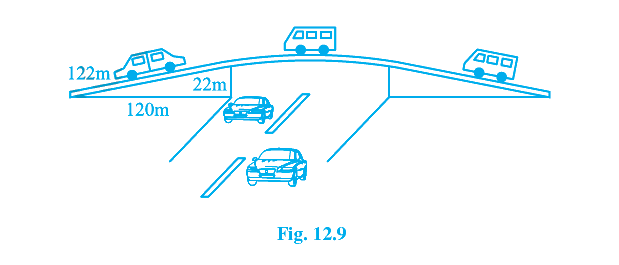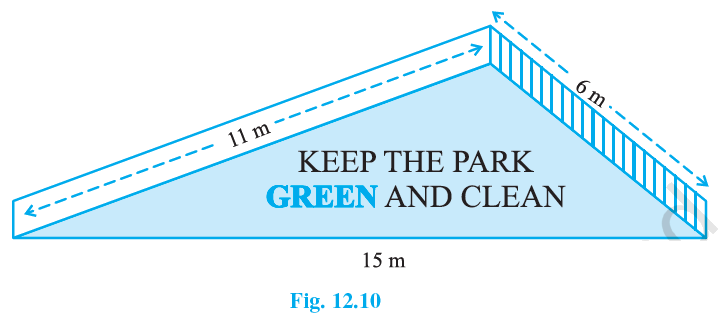# Exercise E12.1 Heron’s Formula NCERT Solutions Class 9

## Chapter 12 Ex.12.1 Question 1

A traffic signal board, indicating ‘SCHOOL AHEAD’, is an equilateral triangle with side ‘$$a$$’. Find the area of the signal board, using Heron’s formula. If its perimeter is $$180 \;\rm{cm}$$, what will be the area of the signal board?

### Solution

What is known?

Dimensions of the traffic signal board (triangle) and its perimeter.

#### What is Unknown?

Area of the signal board.

Reasoning:

By using Heron’s formula we can calculate the area of triangle.

The formula given by Heron about the area of a triangle

\begin{align}=\sqrt{s(s-a)(s-b)(s-c)}\end{align}

Where $$a, b$$ and $$c$$ are the sides of the triangle, and

\begin{align}s &= \text{Semi-perimeter}\\& = \begin{Bmatrix} \text{Half the Perimeter } \\ \text{ of the triangle} \;\end{Bmatrix} \\&=\frac{(a+b+c)}{2}\end{align}

Steps:

Each side of traffic signal board (triangle)$$=‘a’ \;\rm{cm}$$

Perimeter of traffic signal board (triangle) $$=$$ sum of all the sides

\begin{align} &=a+a+a \\ &=3 a \end{align}

Semi Perimeter

\begin{align} s&=\frac{(a+b+c)}{2}\\&=\frac{a+a+a}{2}\\&=\frac{3 a}{2} \end{align}

By using Heron’s formula,

Area of a triangle

$$=\sqrt{s(s-a)(s-b)(s-c)}$$

Area of a (triangle) traffic signal board

\begin{align}&=\sqrt{s(s-a)(s-b)(s-c)} \\& =\sqrt{s(s-a)(s-a)(s-a)} \\& =(s-a) \sqrt{s(s-a)}\quad....(1)\end{align}

We know \begin{align}s=\frac{3 a}{2}\end{align} so substituting in equation (1)

\begin{align}&{=\left(\frac{3 a}{2}-a\right) \sqrt{\frac{3 a}{2}\left(\frac{3 a}{2}-a\right)}} \\ &{=\left(\frac{a}{2}\right) \sqrt{\frac{3 a}{2}\left(\frac{a}{2}\right)}} \\ &{=\frac{a}{2} \times \frac{a}{2} \sqrt{3}} \\ &{=\frac{a^{2}}{4} \sqrt{3}}\end{align}

Area of the signal board

\begin{align}=\frac{a^{2}}{4} \sqrt{3} \;\; \text{sq. units}\end{align}

Now given perimeter $$=$$ $$180 \;\rm{cm}$$

Each side of triangle

\begin{align}&=\frac{{180}}{3}\,\mathrm{cm} \\ a &={60 \,\mathrm{cm}}\end{align}

Area of the signal board

\begin{align}&={\frac{a^{2}}{4} \sqrt{3}} \\ &={\frac{60^{2}}{4} \sqrt{3}}\end{align}

Area of the signal board

$$=900 \sqrt{3} \;\rm{cm^2}$$

## Chapter 12 Ex.12.1 Question 2

The triangular side walls of a flyover have been used for advertisements. The sides of the walls are $$122$$ m, $$22$$m and $$120$$m see Figure. The advertisements yield an earning of Rs $$5000$$ per sq. m per year. A company hired one of its walls for $$3$$ months. How much rent did it pay?### Solution

What is known?

Dimensions of the triangular sides of walls.

What is Unknown?

Rent to pay.

Reasoning:

By using Heron’s formula we can calculate the area of triangle.

The formula given by Heron about the area of a triangle

$$=\sqrt{s(s-a)(s-b)(s-c)}$$

Where $$a, b$$ and $$c$$ are the sides of the triangle, and

\begin{align}s &= \text{Semi-perimeter} \\ & = \begin{Bmatrix} \text{ Half the Perimeter } \\ \text{of the triangle} \end{Bmatrix} \\ & =\frac{(a+b+c)}{2}\end{align}

Steps:

Triangular sides of walls are:

$$a= 122 \;{\rm{m}} , b = 22 \;{\rm{m}}, c = 120\;\rm{ m}$$

Semi Perimeter

\begin{align}s &=\frac{(a+b+c)}{2} \\ &=\frac{122+22+120}{2} \\ &=\frac{264}{2} \\&=132 \; \rm{m}\end{align}

By using Heron’s formula,

Area of a triangle

$$=\sqrt{s(s-a)(s-b)(s-c)}$$

Area of triangular wall

\begin{align}&=\sqrt{s(s-a)(s-b)(s-c)} \\ &=\!\sqrt{\!132(132\!-\!122) (132\!-\!22) (132\!-\!120)} \\& =\sqrt{132 \times 10 \times 110 \times 12} \\ &=\sqrt{132 \times 10 \times 132 \times 10} \\ &=1320 \,\mathrm{m}\end{align}

Rent of $$1\;\rm{m^2}$$ area per year$$=\rm{Rs}\;5000$$

Rent of $$1 \;\rm{m^2}$$ area per month $$= \rm{Rs}\; \frac{5000}{12}$$

Rent of  $$1320$$ $$\mathrm{m}^{2}$$ area for  $$3$$ months

\begin{align} &=\operatorname{Rs} \frac{5000}{12} \times 3 \times 1320 \\ &=\mathrm{Rs} .1650000 \end{align}

Therefore, the rent to pay is  $$\rm{Rs}\;1650000$$.

## Chapter 12 Ex.12.1 Question 3

There is a slide in a park. One of its side walls has been painted in some colour with a message “KEEP THE PARK GREEN AND CLEAN” see Figure.  If the sides of the wall are $$15$$m, $$11$$m and $$6$$m, find the area painted in colour.### Solution

What is known?

Sides of the wall i.e. Dimensions of the triangle.

What is unknown?

Area of the (triangle) i.e. area of slope painted in colour.

Reasoning:

By using Heron’s formula we can calculate the area of triangle.

The formula given by Heron about the area of a triangle

$$=\sqrt{s(s-a)(s-b)(s-c)}$$

Where $$a, b$$ and $$c$$ are the sides of the triangle, and

\begin{align}s &= \text{Semi-perimeter}\\& = \begin{Bmatrix} \text{Half the Perimeter } \\ \text{ of the triangle} \;\end{Bmatrix} \\&=\frac{(a+b+c)}{2}\end{align}

Steps:

The sides of the walls (triangle) are $$a =11\; {\rm{m}} , b = 6 \, {\rm{m}}\;\text{and} \; c=15\, {\rm{m}}.$$

Semi Perimeter:

\begin{align}s & =\frac{(a+b+c)}{2} \\ & =\frac{11+6+15}{2} \\ &=\frac{32}{2} \\ &=16 \mathrm{m}\end{align}

By using Heron’s formula,

Area of a triangle

$$=\sqrt{s(s-a)(s-b)(s-c)}$$

Area of a triangle wall:

\begin{align}&=\sqrt{s(s-a)(s-b)(s-c)} \\ &=\!\sqrt{\!16(16\!-\!11)(16\!-\!6) (16\!-\!15) } \\ &=\sqrt{16 \times 5 \times 10 \times 1} \\ &=\sqrt{800} \mathrm{m}^{2} \\ &=20 \sqrt{2} \mathrm{m}^{2}\end{align}

Area of the wall of park painted in color $$= 20 \sqrt{2} \;\rm{m^2}$$

## Chapter 12 Ex.12.1 Question 4

Find the area of a triangle two sides of which are $$18\;\rm{cm}$$ and $$10\;\rm{cm}$$ and the perimeter is $$42\;\rm{cm}$$.

### Solution

What is known?

Two sides of the triangle and its perimeter.

What is unknown?

Area of the triangle and one of its side.

Reasoning:

By using Heron’s formula we can calculate the area of triangle.

The formula given by Heron about the area of a triangle

$$=\sqrt{s(s-a)(s-b)(s-c)}$$

Where $$a, b$$ and $$c$$ are the sides of the triangle, and

\begin{align}s &= \text{Semi-perimeter}\\& = \begin{Bmatrix} \text{Half the Perimeter } \\ \text{ of the triangle} \;\end{Bmatrix} \\&=\frac{(a+b+c)}{2}\end{align}

Steps:

The sides of triangle given: $$a =18 \, {\rm{cm}} , b = 10 \, {\rm{cm}}$$

Perimeter of the triangle

\begin{align} &= a + b + c\\ {42}&={18+10+c} \\ {42}&={28+c} \\ {c}&={42-28} \\ {c}&={14}\end{align}

Semi Perimeter

\begin{align}s & =\frac{(a+b+c)}{2}\\ &=\frac{42}{2} \\ &=21 \mathrm{cm}\end{align}

By using Heron’s formula,

Area of a triangle

\begin{align}&={\sqrt{s(s-a)(s-b)(s-c)}} \\ &=\sqrt{ 21(21-18) (21-10) (21-14) } \\ &=\sqrt{21 \times 3 \times 11 \times 7} \\ &=21 \sqrt{11} \mathrm{cm}^{2}\end{align}

Area of a triangle $$=21\sqrt{11}\mathrm{cm}^{2}$$

## Chapter 12 Ex.12.1 Question 5

Sides of a triangle are in the ratio of $$12:17:25$$ and its perimeter is $$540\; \rm{cm}$$. Find its area.

### Solution

What is known?

Ratio of sides of the triangle and its perimeter.

What is unknown?

Area of the triangle.

Reasoning:

By using Heron’s formula we can calculate the area of triangle.

The formula given by Heron about the area of a triangle

$$=\sqrt{s(s-a)(s-b)(s-c)}$$

Where $$a, b$$ and $$c$$ are the sides of the triangle, and

\begin{align}s &= \text{Semi-perimeter}\\& = \begin{Bmatrix} \text{Half the Perimeter } \\ \text{ of the triangle} \;\end{Bmatrix} \\&=\frac{(a+b+c)}{2}\end{align}

Steps:

Suppose the sides are $$12x\;\rm {cm}$$, $$17x\;\rm {cm}$$ and $$25x\rm\; {cm}$$.

Perimeter of the triangle $$=$$ $$540\; \rm{cm}$$

\begin{align}{12 x+17 x+25 x=540} \\ {54 x=540} \\ {x=\frac{540}{54}} \\ {x=10 \mathrm{cm}}\end{align}

Therefore sides of triangle:

\begin{align}&12 x=12 \times 10=120 \;\mathrm{cm},\\ & 17 x=17 \times 10=170 \;\mathrm{cm}, \\ & 25 x=25 \times 10=250 \;\mathrm{cm}\end{align}

$$a = 120\; {\rm{cm}} ,\\ b = 170\;{\rm{cm}},\\ c = 250\;{\rm{cm}}$$

\begin{align}s &= \text{Half the Perimeter}\\ s&=\frac{540}{2}\\&=270 \;\mathrm{cm}\end{align}

By using Heron’s formula,

Area of a triangle

\begin{align}&={\sqrt{s(s-a)(s-b)(s-c)}} \\&=\!\sqrt{270(270\!-\!120)\!(270\!-\!170)\!(270\!-\!250)}\\ &=\sqrt{270 \times 150 \times 100 \times 20} \\ &=\sqrt{81000000} \\ &=9000 \mathrm{cm}^{2}\\\end{align}

Area of a triangle $$=$$$$9000 \;\rm{cm^2}.$$

## Chapter 12 Ex.12.1 Question 6

An isosceles triangle has perimeter $$30\; \rm{cm}$$ and each of the equal sides is $$12 \;\rm{cm}$$. Find the area of the triangle.

### Solution

What is known?

Equal Sides of the triangle and its perimeter.

What is unknown?

Area of the triangle.

Reasoning:

By using Heron’s formula we can calculate the area of triangle.

The formula given by Heron about the area of a triangle

$$=\sqrt{s(s-a)(s-b)(s-c)}$$

Where $$a, b$$ and $$c$$ are the sides of the triangle, and

\begin{align}s &= \text{Semi-perimeter}\\& = \begin{Bmatrix} \text{Half the Perimeter } \\ \text{ of the triangle} \;\end{Bmatrix} \\&=\frac{(a+b+c)}{2}\end{align}

Steps:

Equal sides: $$a = b =12 \rm{cm}$$

\begin{align}\text{Perimeter}&={a+b+c} \\ 30 &=12+12+c \\ c &=30-24 \\ c &=6 \;\mathrm{cm} \end{align}

\begin{align}\text{Semi Perimeter s}&=\frac{(a+b+c)}{2}\\&=\frac{30}{2}\\&=15 \,\mathrm{cm}\end{align}

By using Heron’s formula,

Area of a triangle

\begin{align}&={\sqrt{s(s-a)(s-b)(s-c)}} \\ &=\sqrt{ 15(15-12) (15-12) (15-6) } \\ &=\sqrt{15 \times 3 \times 3 \times 9} \\ &=9 \sqrt{15} \;\mathrm{cm}^{2}\end{align}

Area of a triangle $$= 9 \sqrt{15} \;\mathrm{cm}^{2}$$

Instant doubt clearing with Cuemath Advanced Math Program